International
Tables for
Crystallography
Volume A1
Symmetry relations between space groups
Edited by Hans Wondratschek and Ulrich Müller

International Tables for Crystallography (2006). Vol. A1, ch. 1.4, pp. 27-28   | 1 | 2 |
https://doi.org/10.1107/97809553602060000540

## Chapter 1.4. Computer checking of the subgroup data

Franz Gählera*

aInstitut für Theoretische und Angewandte Physik, Universität Stuttgart, D-70550 Stuttgart, Germany
Correspondence e-mail: gaehler@itap.physik.uni-stuttgart.de

Most of the data in Part 2of this volume were checked by a computer-algebra program based upon the package Cryst, which in turn is an extension of the computer-algebra program GAP. The basic capabilities of Cryst are described. A space group is represented as a group of augmented matrices. The implementation of a membership test for such groups is explained, along with how the test can be used to determine whether two space groups are equal or whether one is a subgroup of the other. The algorithm for computing the maximal subgroups of space groups is also outlined. The tests that were performed are then described. The program parsed thesource files that were used to produce the tables of data. All the data tabulated for the low-index subgroups were checked for correctness and consistency. The low-index subgroups were recomputed from scratch and compared with the tabulated subgroups. Unfortunately, such tests could not be applied to the infinite series of maximal isomorphic subgroups of arbitrarily high index.

### 1.4.1. Introduction

| top | pdf |

Most of the data in Part 2of this volume have been checked by a computer-algebra program. This program is built upon the package Cryst (Eick et al., 2001), formerly known as CrystGAP (Eick et al., 1997), which in turn is an extension of the computer-algebra program GAP (The GAP Group, 2002). GAP is a program system for computations involving general algebraic structures, in particular groups. Since space groups are infinite groups, the generic algorithms for finite groups often cannot be used for space groups. The Cryst package provides the special methods and algorithms needed for working with space groups, thereby extending the field of applicability of GAP. The algorithms used in Cryst have been described by Eick et al. (1997).

### 1.4.2. Basic capabilities of the Cryst package

| top | pdf |

Before we describe in more detail the checks that have been performed, we briefly summarize the capabilities of the Cryst package and how space groups are handled by Cryst.

In Cryst, a space group is represented as a fixed group of augmented matrices, cf. Section 1.2.2.4. The group multiplication therefore coincides with matrix multiplication. The space group is defined by an arbitrary (finite) set of generating elements. The space group consists of the set of all augmented matrices that can be obtained as products of the generating matrices. It is not important which generating elements are chosen. Different sets of generators can define the same space group.

The representation of a space group as a group of augmented matrices implicitly requires the choice of an origin and a vector basis of Euclidean space. The Cryst package does not require any particular choice of origin or vector basis. In particular, it is not necessary to work with a lattice basis; in fact, any basis can be used. This has the advantage that one can continue to use the basis chosen for the parent group when passing to a subgroup.

Space groups are conjugated by some affine coordinate transformation if they differ only in their settings, i.e. by the choice of their origins and/or vector bases. Such an affine coordinate transformation is also represented by an augmented matrix. Given a coordinate transformation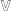and a space group, the transformed space group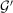is simply generated by the transformed generators of.

If a space groupis given by a set of generators, Cryst first needs to compute the point group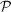of, and the translation subgroup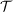, represented by a (canonical) basis of the translation lattice (the basis of the translation lattice chosen by Cryst is canonical in the sense that the same basis is always chosen for a given). The point groupis simply generated by the linear parts of the generators of. The mapping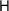, which sends each element ofto its linear part, is in fact a group homomorphism: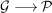. The point groupis finite and each point-group element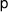can easily be expressed as a product of generators of, which are images of generators ofunder. This provides a way to compute, for any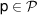, some representative pre-image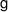ofunder, i.e. some elementwhich is mapped ontoby. Having expressed the elementas a product of generators, one simply replaces the factors in this product by their representative pre-images under, which are known by construction, to obtain the representative pre-imageof.

To compute a generating set of the translation subgroup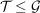, one first computes a set of defining relations of the point groupfor the given generators. For a finite group this is a standard task in GAP. These defining relations are a set of inequivalent ways to express the identity element ofby the generators of. Replacing the factors in such a defining relation by their representative pre-images inyields a pre-image of the identity of, i.e. an element of. The translations so obtained generate, together with the pure translation generators of, the entire translation subgroup.

We now have all the necessary information to test whether a given augmented matrix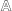is an element of a space group. One first tests whether the linear part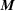ofis an element of the point groupof. If,is not an element of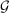. Otherwise, some pre-image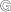ofinis computed.is then an element ofif and only ifanddiffer in their translation part by an element of the translation group. With this membership test, we can determine whether two space groups are equal, or whether one is a subgroup of the other: a space group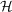is a subgroup of a space groupif all the generators ofare elements of. Two space groups are equal if either of them is a subgroup of index 1 of the other.

### 1.4.3. Computing maximal subgroups

| top | pdf |

The Cryst package has built-in facilities for computing the maximal subgroups of a given index for any space group. More precisely, given a prime number p, Cryst can compute conjugacy-class representatives of those maximal subgroups ofwhose index inis a power of p. The algorithms used for this task are described in Eick et al. (1997). Essentially, one determines the maximal subgroups of the (finite) factor group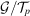, whereis the subgroup of those translations ofwhich are a p-fold multiple of an element of the full translation groupof. After the maximal subgroups are obtained, the translations inare added back to the subgroups.

From a representativeof a conjugacy class of subgroups, the list of all subgroups in the same conjugacy class is obtained by repeatedly conjugating(and the subgroups obtained from it by conjugation) with generators of, until no new conjugated subgroups are obtained. This is also the way of determining whether two subgroups are conjugated: one enumerates the groups in the conjugacy class of one of them, and checks whether the other is among them.

The index of a subgroupofis easily computed as the product of the index of the point group ofin the point group ofand the index of the translation group ofin the translation group of. For maximal subgroups, only one of these factors is different from 1. For klassengleiche subgroups, the two point groups are equal, whereas for translationengleiche subgroups the two translation subgroups are equal. Therefore, a maximal subgroup is easily identified either as a klassengleiche or a translationengleiche subgroup.

### 1.4.4. Description of the checks

| top | pdf |

In order to avoid new errors being introduced in the typesetting process after the data have been checked and corrected, we carried out the computer checks directly on the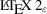sources used for the production of this volume. The tables have been typeset with specially designedmacros, the primary purpose of which was to guarantee a homogeneous layout throughout the book. As a side effect, it was relatively easy to write a GAP program which parses thesources, recognizes the macros, extracts the data (the arguments of the macros), brings the data into a form suitable for further processing with routines from the Cryst package and finally performs the various checks. In this way, the checks could be done fully automatically, and could be repeated after every modification of the tabulated data.

In the following, we describe the checks that have been carried out. We first applied a number of tests individually to each of the tabulated maximal subgroups of low index: whether it is a subgroup, whether the index given is correct, whether the listed coordinate transformation maps the subgroup to the preferred setting of the given space-group type (and thus, whether the space-group type of the subgroup is correct) and whether the listed coordinate transformation maps the given generators of the subgroup to the standard generators of its space-group type, in the same order. Here, the preferred setting is the setting of the parent group, where applicable, and otherwise the preferred setting of the space-group type of the subgroup, if there is more than one setting in the tables.

In a second step, a complete set of maximal subgroups of low index (2, 3 or 4) is computed afresh with the routines from the Cryst package. These subgroups are then divided into conjugacy classes, and classified as klassengleiche or translationengleiche subgroups. This list is then compared with the tabulated list of maximal subgroups. It was verified that each maximal subgroup of a given index was listed exactly once, that the classification into conjugacy classes of subgroups was correct and that the subgroups were correctly identified as klassengleiche or as translationenglei­che subgroups.

All the tests described above concern the maximal subgroups of low index. Unfortunately, similar automatic tests could not be performed on the series of isomorphic subgroups. The subgroups in these series contain variable parameters, and Cryst can only deal with fixed, concrete space groups without free parameters.

### References

Eick, B., Gähler, F. & Nickel, W. (1997). Computing maximal sub-groups and Wyckoff positions of space groups. Acta Cryst. A53, 467–474.Google Scholar
Eick, B., Gähler, F. & Nickel, W. (2001). The `Cryst' Package. Version 4.1. http://www.itap.physik.uni-stuttgart.de/~gaehler/gap/packages.html .Google Scholar
The GAP Group (2002). GAP – Groups, Algorithms and Programming. Version 4.3. http://www.gap-system.org .Google Scholar